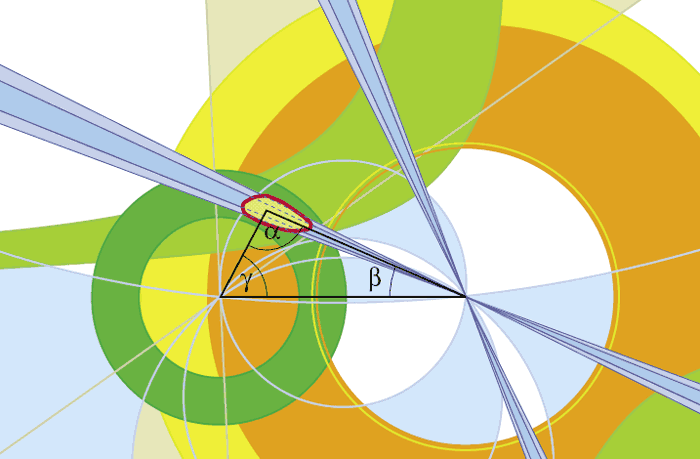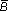A joint Fermilab/SLAC publication

Unitary triangle

01/01/06

The weak force is responsible for the decay of matter: unstable particles made of heavy quarks and antiquarks decay into particles made of their lighter cousins. The rates of these decay processes are related to a set of numbers called the Cabibbo-Kobayashi-Maskawa (CKM) matrix, named for the three physicists who introduced it. The numbers are often shown in a graphical form called the unitarity triangle.

Deconstruction: Unitarity triangle

The weak force is responsible for the decay of matter: unstable particles made of heavy quarks and antiquarks decay into particles made of their lighter cousins. The rates of these decay processes are related to a set of numbers called the Cabibbo-Kobayashi-Maskawa (CKM) matrix, named for the three physicists who introduced it. The numbers are often shown in a graphical form called the unitarity triangle.

The CKM matrix gives rise to CP violation, the subtle difference between matter and antimatter. The area of the triangle is a measure of the amount of CP violation caused by the weak force. This CP violation partly explains why we live in a matter-dominated universe rather than one full of antimatter or radiation.

Experiments at many different laboratories are examining weak decay processes to determine the angles and the lengths of the sides of the triangle. The red blob surrounding the peak of the triangle represents the constraint obtained by combining all measurements: the smaller the blob, the better the physicists’ understanding of the location of the peak, the shape and area of the triangle, and hence the weak force.Graphic source: CKMfitter collaboration The top of the triangle CP violation was first observed in 1964 by studying two particles containing the strange quark: the short-lived KS and the longer-lived KL. In a CP-conserving world, the KS would decay to two particles called pions, and the KL would decay to three. While this is very nearly true, the KL was also found to decay to two pions about 0.1% of the time. This manifestation of CP violation is described by the parameter εK, and measurements of it constrain the peak of the triangle to lie somewhere on the light green, boomerang-shaped region. The angle β By studying the interference between decays of neutral B0 mesons, which consist of an anti-bottom quark and a down quark, and their antiparticles0, physicists determine the angle β of the unitarity triangle. It is the “golden measurement” for the two B factories, BaBar at SLAC in California, and Belle at KEK in Japan. Because what they measure is sin(2β), there are actually four possible solutions for the angle, shown as the four blue jets radiating from the bottom-right corner of the triangle. The other angles: α and γ These two angles are more difficult to measure than β. Quantum effects, so-called “penguin” processes, interfere with direct measurements of α, while measurements of γ require studies of rare decay processes. The B factories have been able to measure these angles, adding two constraints to the triangle. Like β, the angle α produces four allowed regions for the upper vertex, shown as blue arc-shaped areas. The angle γ constrains the location of the peak of the triangle to the two wedges radiating from the bottom-left corner of the triangle. The triangle’s left side Measuring the rate at which bottom quarks decay into up and charm quarks, physicists determine two elements of the CKM matrix called Vub and Vcb. The ratio of these two elements gives the length of the left side of the triangle, the end of which must lie in the dark green circle. Many experiments have measured Vub and Vcb, and the current best measurements come from the B factories at SLAC and KEK. The triangle’s right side The B0 meson can spontaneously turn into a0 meson, its antiparticle. The rate at which this transformation occurs has been measured by a number of experiments and constrains the length of the right side of the triangle, placing its end in the yellow ring (largely covered by the orange ring). Studying the Bs meson, which contains an anti-bottom quark and a strange quark, and finding no evidence for its transformation into the antiparticles, physicists know that the end point of the right side must lie within the orange ring only. Experiments at Fermilab are currently improving this measurement, and may soon observe Bs tos mixing for the first time.

Looking for new physics
Currently, all measurements of the unitarity triangle are consistent with the peak lying somewhere within the red-outlined region. By improving the current measurements and performing new ones, scientists will reduce the size of this allowed region, measuring the position of the vertex ever more precisely. If any experimental result is inconsistent with this vertex, scientists have evidence that the CKM picture of the weak force is incomplete. Such a discovery would overhaul our current understanding of the weak force, and provide us with a glimpse of new physics that may have played a role in the evolution of the early universe.

Michael Mazur, Istituto Nazionale di Fisica Nucleare (INFN), Pisa